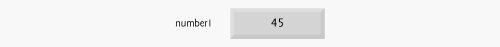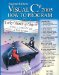# Memory Concepts

Variable names such as number1, number2 and sum actually correspond to locations in the computer's memory. Every variable has a name, a type, a size and a value.

In the addition application of Fig. 3.18, when the statement (line 16)

```number1 = Convert.ToInt32( Console.ReadLine() );
```

executes, the number typed by the user is placed into a memory location to which the name number1 has been assigned by the compiler. Suppose that the user enters 45. The computer places that integer value into location number1, as shown in Fig. 3.19. Whenever a value is placed in a memory location, the value replaces the previous value in that location and the previous value is lost.

Figure 3.19. Memory location showing the name and value of variable number1.When the statement (line 20)

```number2 = Convert.ToInt32( Console.ReadLine() );
```

executes, suppose that the user enters 72. The computer places that integer value into location number2. The memory now appears as shown in Fig. 3.20.

Figure 3.20. Memory locations after storing values for number1 and number2.After the application of Fig. 3.18 obtains values for number1 and number2, it adds the values and places the sum into variable sum. The statement (line 22)

```sum = number1 + number2; // add numbers
```

performs the addition, then replaces sum's previous value. After sum has been calculated, memory appears as shown in Fig. 3.21. Note that the values of number1 and number2 appear exactly as they did before they were used in the calculation of sum. These values were used, but not destroyed, as the computer performed the calculationwhen a value is read from a memory location, the process is nondestructive.

Figure 3.21. Memory locations after calculating and storing the sum of number1 and number2.Visual C# 2005 How to Program (2nd Edition)
ISBN: 0131525239
EAN: 2147483647
Year: 2004
Pages: 600